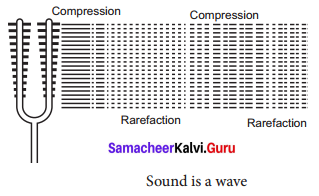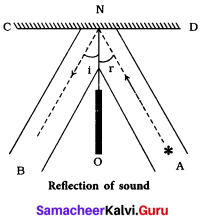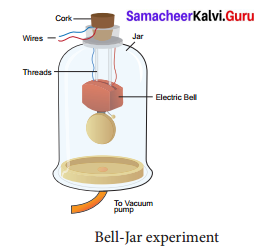# Samacheer Kalvi Books: Tamilnadu State Board Text Books Solutions

## Samacheer Kalvi 9th Science Chapter 8 Sound Notes PDF Download: Tamil Nadu STD 9th Science Chapter 8 Sound NotesSamacheer Kalvi 9th Science Chapter 8 Sound Notes PDF Download: Tamil Nadu STD 9th Science Chapter 8 Sound Notes

We bring to you specially curated Samacheer Kalvi 9th Science Chapter 8 Sound Notes PDF which have been prepared by our subject experts after carefully following the trend of the exam in the last few years. The notes will not only serve for revision purposes, but also will have several cuts and easy methods to go about a difficult problem.

 Board Tamilnadu Board Study Material Notes Class Samacheer Kalvi 9th Science Subject 9th Science Chapter Chapter 8 Sound Format PDF Provider Samacheer Kalvi Books

## How to Download Samacheer Kalvi 9th Science Chapter 8 Sound Notes PDFs?

2. Click on the Samacheer Kalvi 9th Science Notes PDF.
3. Look for your preferred subject.
4. Now download the Samacheer Kalvi 9th Science Chapter 8 Sound notes PDF.

## Download Samacheer Kalvi 9th Science Chapter 8 Sound Chapterwise Notes PDF

1. amplitude
2. ECG
3. reverberation

III. Match the following.

 Column – I Column – II (a) Tuning fork (i) The point where density of air is maximum (b) Sound (ii) Maximum displacement from the equilibrium position (c) Compressions (iii) The sound whose frequency is greater than 20,000 Hz (d) Amplitude (iv) Longitudinal wave (e) Ultrasonics (v) Production of sound

(a) (v)
(b) (i)
(c) (iv)
(d) (ii)
(e) (iii)

Question 1.
Through which medium sound travels faster, iron or water? Give reason.
Sound travels faster through iron as solids are packed together tighter than liquids and gases.

Question 2.
Name the physical quantity whose SI unit is ‘hertz’. Define.
The SI unit of frequency is hertz (Hz). The number of vibrations (complete waves or cycles) produced in one second is called frequency of the wave.

Question 3.
What is meant by supersonic speed?
When the speed of any object exceeds the speed of sound in air (330 m s-1) it is said to be travelling at supersonic speed.

Question 4.
How does the sound produced by a vibrating object in a medium reach your ears?
When an object vibrates, it forces the neighbouring particles of the medium to vibrate. These vibrating particles then force the particles adjacent to them to vibrate. In this way vibrations produced by an object are transferred till it reaches the ear.

Question 5.
You and your friend are on the moon. Will you be able to hear any sound produced by your friend?
No, as there is no medium on moon for the sound to travel.

Question 1.
Describe with diagram, how compressions and rarefactions are produced.
Sound is also a longitudinal wave. Sound can travel only when there are particles which can be compressed and rarefied. Compressions are the regions where particles are crowded together. Rarefactions are the regions of low pressure where particles are spread apart. A sound wave is an example of a longitudinal mechanical wave. Below figure represents the longitudinal nature of sound wave in the medium.Question 2.
Verify experimentally the laws of reflection of sound.
The laws of reflection are:

• The angle in which the sound is incident is equal to the angle in which sound is reflected.
• Direction of incident sound, direction of the reflected sound and the normal are in the same plane.Take two metal tubes A and B. Keep one end of each tube on a metal plate as shown in figure. Place a wrist watch c at the open end of the tube A and interpose a cardboard between A and B. Now at a particular inclination of the tube B with the cardboard, ticking of the watch is clearly heard. The angle of reflection made by the tube B with the cardboard is equal to the angle of incidence made by the tube A with the cardboard.
For example, if the angle of incidence is 20° on the left side of a protractor (see figure arrangement), the angle of reflection at which we are able to hear the sound clearly will also be at 20° on the right side of the protractor.
∴ ∠ i = ∠ r    ➝ verifying Law I
We will also further observe that pipe 1, pipe 2 i.e, the incident ray, reflected ray and the normal lie on the same plane, verifying law II.

Question 3.
List the applications of sound.
Applications of ultrasound

• Ultra sound can be used in cleaning technology. Minute foreign particles can be removed from objects placed in a liquid bath through which ultrasound is passed.
• Ultrasounds can also be use d to detect cracks and flaws in metal blocks.
• Ultrasonic waves are made to reflect from various parts of the heart and form the image of the heart. This technique is called ‘echo cardiography’.
• Ultrasound may be employed to break small ‘stones’ formed in the kidney into fine grains. These grains later get flushed out with urine.

Question 4.
Explain how does SONAR work?
SONAR stands for Sound Navigation And Ranging. Sonar is a device that uses ultrasonic waves to measure the distance, direction and speed of underwater objects. Sonar consists of Science – 9 (Physics)
a transmitter and a detector and is installed at the bottom of boats and ships. The transmitter produces and transmits ultrasonic waves. These waves travel through water and after striking the object on the seabed, get reflected back and are sensed by the detector.

The detector converts the ultrasonic waves into electrical signals which are appropriately interpreted. The distance of the object that reflected the sound wave can be calculated by knowing the speed of sound in water and the time interval between transmission and reception of the ultrasound. Sonar technique is used to determine the depth of the sea and to locate underwater hills, valleys, submarine, icebergs etc.

VI. Numerical problem.

Question 1.
The frequency of a source of sound is 600 Hz. How many times does it vibrate in a minute?
The number of vibrations (complete waves or cycles) produced in one second is called frequency of the wave.
Hence, in one minute 600 x 60 vibrations are produced = 36000 (Need clarification)

Question 2.
A stone is dropped from the top of a tower 750 m high into a pond of water at the base of the tower. When is the splash heard at the top?
(Given g = 10 m s– 2 and speed of sound = 340 ms– 1)
Height = 750m
h = ut + 0.5 gt2
The initial velocity is 0
750 = 0.5 × 10 × t2
t = 10sec
Speed of sound is 340m/sec
So, time taken to travel 750m upwards is,
750340 = 2.20s
time taken = 10 + 2.20 = 12.2 sec.

### Samacheer Kalvi 9th Science Sound In Text Problems

Question 1.
A sound wave has a frequency of 2 kHz and wavelength of 15 cm. How much time will it take to travel 1.5 km?
Solution:
v = nλ
n = 2 kHz = 2000Hz
λ = 15 cm = 0.15 m
0.15 × 2000 = 300ms– 1
Time(t) =  Distance (d)  Velocity (v)
The sound will take 5 s to travel a distance of 1.5 km.

Question 2.
What is the wavelength of a sound wave in air at 20° C with a frequency of 22 MHz?
Solution:
λ = v/n
Here, v = 344 m s– 1
n = 22 MHz = 22 × 106 Hz
λ = 34422 × 106 = 15.64 × 106 m = 15.64 µm.

Question 3.
A man fires a gun and hears its echo after 5 s. The man then moves 310 m towards the hill and fires his gun again. If he hears the echo after 3 s, calculate the speed of sound.
Solution:
Distance (d) = velocity (v) × time (t)
Distance travelled by sound when gun fires first time, 2d = v × 5 …………. (1)
Distance travelled by sound when gun fires second time, 2d – 620 = v × 3 …………… (2)
Rewriting equation (2) as,
2d = (v × 3) + 620 ……………. (3)
Equating (1) and (3), 5v = 3v + 620
2v = 620 .
Velocity of sound, v = 310 m s– 1

Question 4.
A ship sends out ultrasound that returns from the seabed and is detected after 3.42 s.
If the speed of ultrasound through sea water is 1531m s– 1, what is the distance of the seabed from the ship?
Solution:
We know, distance = speed × time
2d = speed of ultrasound × *time
2d = 1531 × 3.42
∴ d = 52362 = 2618 m
Thus, the distance of the seabed from the ship is 2618 m or 2.618 km.

### Samacheer Kalvi 9th Science Sound Additional Questions

Question 1.
“Sound needs a medium for propagation”. Justify with an experiment.Sound needs a material like air, water, steel, etc. for its propagation. It cannot travel through vacuum. This can be demonstrated by the Bell-Jar experiment.

An electric bell and an airtight glass jar are taken. The electric bell is suspended inside the airtight jar. The jar is connected to a vacuum pump. If the bell is made to ring, we will be able to hear the sound of the bell. Now when the jar is evacuated with the vacuum pump, the air in the jar is pumped out gradually and the sound becomes feebler and feebler. We will not hear any sound, if the air is fully removed (if the jar has vacuum).

Question 2.
Name the characteristics that describe a sound.
A sound wave can be described completely by five characteristics viz – amplitude, frequency, time period, wavelength and velocity or speed.

Question 3.
What does the intensity of sound heard at a place depend on?
The intensity of sound heard at a place depends on the following factors;

1. Amplitude of the source
2. Distance of the observer
3. Surface area of the source
4. Density of the medium
5. Frequency of the source.

Question 4.
Why is sound wave called longitudinal wave?
As the vibration of the particles of the medium are along the direction of wave propagation, sound waves are longitudinal waves.

Question 5.
What is sound and how is it produced?
Sound is a form of energy which produces the sensation of hearing. It is produced due to vibrations of different objects, eg. stretched vibrating wires and vibrating drums, etc.

Question 6.
How can two whales in the sea, hundreds of kilometers apart, communicate?
Sound travels about 5 times faster in water than in air. Therefore, two whales in the sea, hundreds of kilometers apart can communicate easily.

Question 7.
What is a stethescope? What is the principle on which it works?
Stethescope is a medical instrument used for listening to sounds produced in the body. In stethescopes, the sounds reach doctor’s ears by multiple reflections that happen in the connecting tube.

Question 8.
What is an ECG? How does it help in the field of medicine?
The electrocardiogram (ECG) is one of the simplest and oldest cardiac investigations available. In ECG, the sound variations produced by heart is converted into electric signals. Thus an ECG is simply a representation of the electrical activity of the heart muscle as it changes with time. Usually it is printed on paper for easy analysis. The sum of this electrical activity, when amplified and recorded for just a few seconds is known as an ECG.

## How to Prepare using Samacheer Kalvi 9th Science Chapter 8 Sound Notes PDF?

Students must prepare for the upcoming exams from Samacheer Kalvi 9th Science Chapter 8 Sound Notes PDF by following certain essential steps which are provided below.

• Use Samacheer Kalvi 9th Science Chapter 8 Sound notes by paying attention to facts and ideas.
• Pay attention to the important topics
• Refer TN Board books as well as the books recommended.
• Correctly follow the notes to reduce the number of questions being answered in the exam incorrectly
• Highlight and explain the concepts in details.

## Frequently Asked Questions on Samacheer Kalvi 9th Science Chapter 8 Sound Notes

#### How to use Samacheer Kalvi 9th Science Chapter 8 Sound Notes for preparation??

Read TN Board thoroughly, make separate notes for points you forget, formulae, reactions, diagrams. Highlight important points in the book itself and make use of the space provided in the margin to jot down other important points on the same topic from different sources.

#### How to make notes for Samacheer Kalvi 9th Science Chapter 8 Sound exam?

Read from hand-made notes prepared after understanding concepts, refrain from replicating from the textbook. Use highlighters for important points. Revise from these notes regularly and formulate your own tricks, shortcuts and mnemonics, mappings etc.
Share: